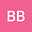Convergence Analysis of Legendre wavelets in numerical solution of linear weakly singular Volterra integral equation for union of some intervals with application in heat conduction
•Abstract

In this paper we apply the Legendre wavelets basis to solve the linear weakly singular Volterra integral equation of the second kind. The basis is defined on [0,1) , and in this work we extend this interval to [0,n) for some positive integer n. For this aim we solve the problem on [0,1); then we apply the Legendre wavelets on [1,2) and use the lag solution on [0,1) to obtain the solution on [0,2) and continue this procedure. Convergence analysis of Legendre wavelets on [n,n+1), is considered in Section2. We give a convergence analysis for the proposed method, established on compactness of operators. In numerical results we give two sample problems from heat conduction. For this purpose, in Section 6 we give an equivalent theorem between the proposed heat conduction problem and an integral equation. Then we solve the equivalent integral equation by the proposed method on union of some interval and obtain the solution of the heat conduction problem. As Tables and Figures of two and three dimensional plots show, accuracy of the method is reasonable and there is not any propagation of error from lag intervals. The convergence analysis and these sample problems demonstrate the accuracy and applicability of the method.

Peer review status:UNDER REVIEW

20 Nov 2021Submitted to Mathematical Methods in the Applied Sciences
25 Nov 2021Assigned to Editor
25 Nov 2021Submission Checks Completed
27 Nov 2021Reviewer(s) Assigned
19 Dec 2021Review(s) Completed, Editorial Evaluation Pending